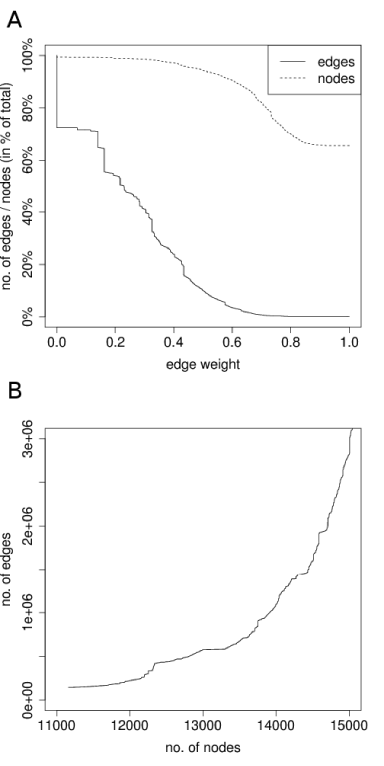Figure 3: (A) Edge weight distribution and node coverage as a function of edge weight. Node and edge count are presented for each weight cutoff in % with respect to the entire set of nodes and theoretical edges, with a start number of nodes of 11,162 at an edge weight of 1.0 (145,391 edges as extracted from experimentally verified interaction data sources), and a maximum number of nodes being 17,022 with a maximum number of edges when considering all node pairs being about 140 million. (B) Relation of node and edge count at the different edge weight cutoff values, starting at an edge weight of 1.0 (with 11,162 nodes).